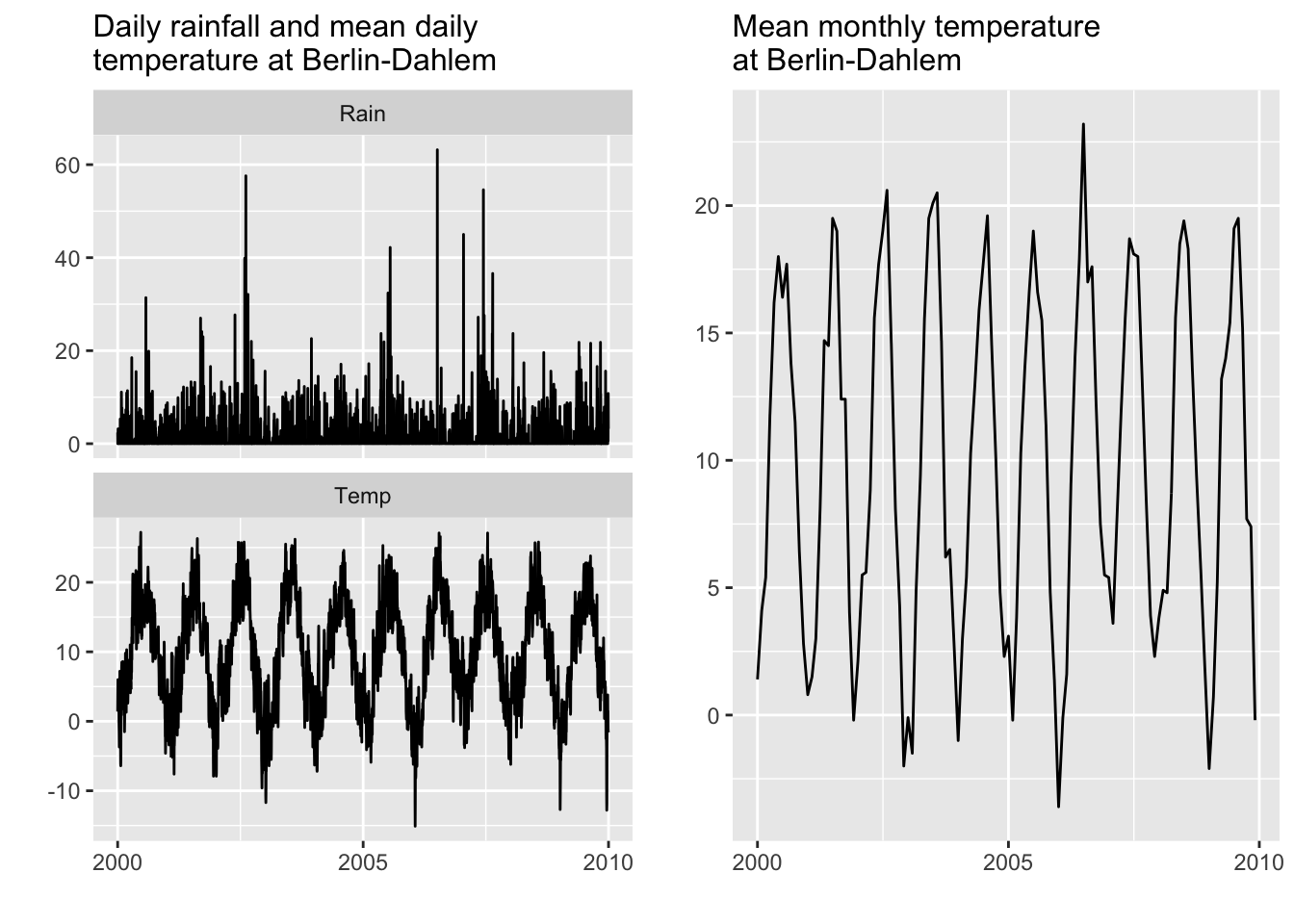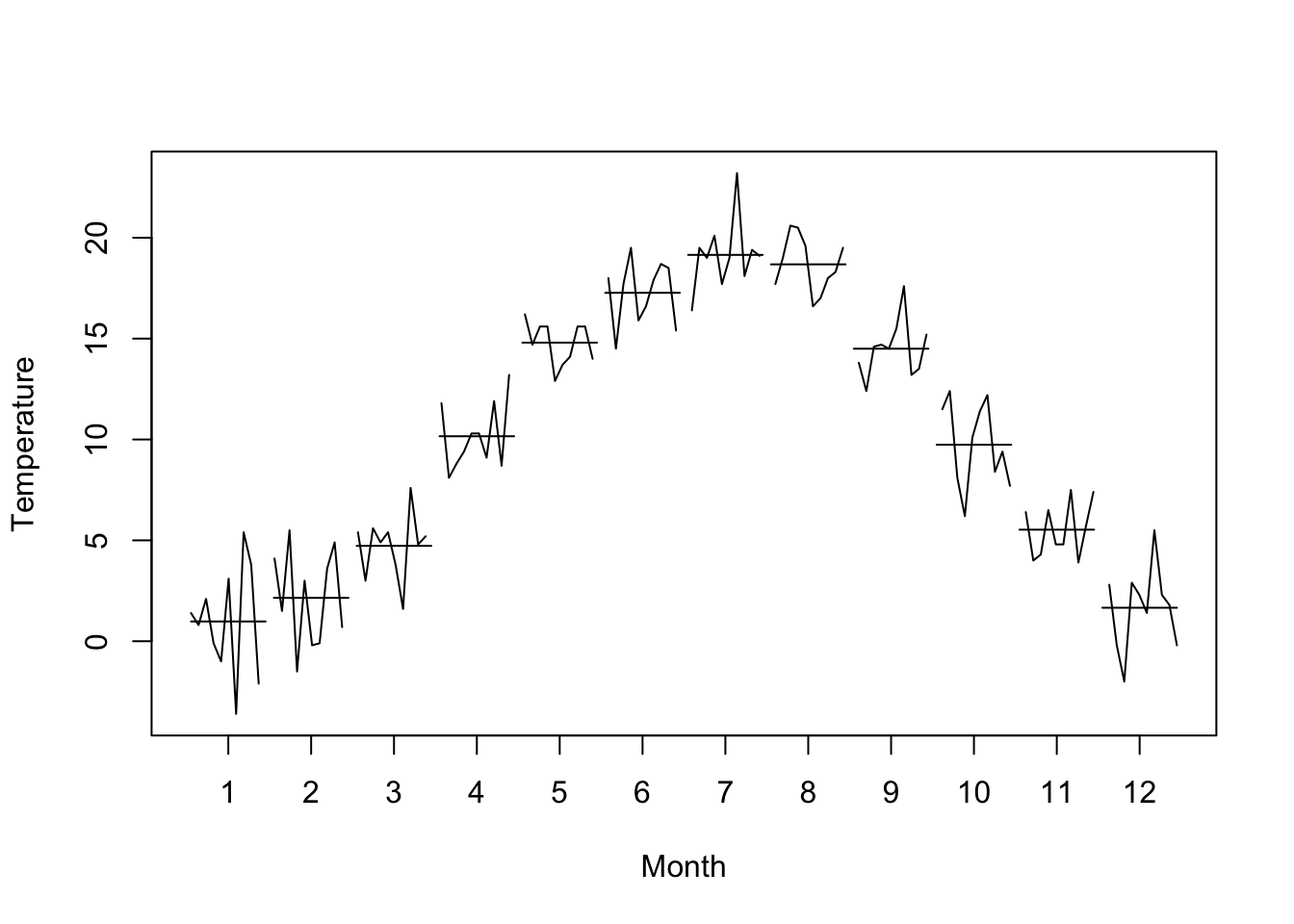A very important and recurrent task in time series analysis is the calculation of summary statistics. In order to introduce summary statistics for time series analysis we revisit the monthly, daily and hourly data sets from the weather station Berlin-Dahlem (FU). Check out the previous section on Data sets used to remind yourself how we processed the data.

Let us load the monthly (`ts.FUB.monthly`), daily (`ts.FUB.daily`) and hourly (`ts.FUB.hourly`) time series data for the weather station Berlin-Dahlem (FU) into R. Therefore we apply the `load()` function.

``load(url("https://userpage.fu-berlin.de/soga/300/30100_data_sets/DWD_FUB.RData"))``

For the sake of simplicity we reduce the monthly and daily data sets and focus on the 10-year period from 2000 to 2009.

``````library(xts)
library(lubridate)
library(ggfortify)
library(gridExtra)

### 10-year period from 2000 to 2009 daily data ###
daily.2000.2009 <- ts.FUB.daily['2000/2009', ]

### 10-year period from 2000 to 2009 monthly data ###
monthly.2000.2009 <- ts.FUB.monthly['2000/2009']

### PLOTTING ###
p1 = autoplot(daily.2000.2009,
main = 'Daily rainfall and mean daily \ntemperature at Berlin-Dahlem') +
theme(plot.title = element_text(size = 12))

p2 = autoplot(monthly.2000.2009,
main = 'Mean monthly temperature \nat Berlin-Dahlem') +   theme(plot.title = element_text(size = 12))

grid.arrange(p1, p2, ncol = 2) ``````To get a quick overview on the statistical characteristics of time series we use the `summary()` function. The function returns basic statistics for the whole data set.

``summary(monthly.2000.2009)``
``````##      Index            monthly.2000.2009
##  Min.   :2000-01-01   Min.   :-3.600
##  1st Qu.:2002-06-23   1st Qu.: 4.075
##  Median :2004-12-16   Median : 9.750
##  Mean   :2004-12-15   Mean   : 9.946
##  3rd Qu.:2007-06-08   3rd Qu.:15.675
##  Max.   :2009-12-01   Max.   :23.200``````
``summary(daily.2000.2009)``
``````##      Index                 Temp              Rain
##  Min.   :2000-01-01   Min.   :-15.100   Min.   : 0.000
##  1st Qu.:2002-07-02   1st Qu.:  4.000   1st Qu.: 0.000
##  Median :2004-12-31   Median : 10.300   Median : 0.000
##  Mean   :2004-12-31   Mean   :  9.976   Mean   : 1.684
##  3rd Qu.:2007-07-02   3rd Qu.: 16.000   3rd Qu.: 1.500
##  Max.   :2009-12-31   Max.   : 27.200   Max.   :63.200``````

Another useful function is the `monthplot()` function, which plots seasonal (monthly by default) sub-series of a time series. For each season (month) a time series is plotted and a defined function, such as the mean (default), the median or the standard deviation, among others, is applied to the sub-series. The default method assumes observations come in groups of 12.

``````monthplot(monthly.2000.2009,
ylab = 'Temperature',
xlab = 'Month')``````The horizontal bars represent the mean monthly temperature and the lines represent the time series for each particular sub-series (month). A more recent implementation of the same logic is provided by the `ggfreqplot()` function, which allows us to specify a confidence interval. To showcase the function we first subset the monthly time series to the 20th century by using the `window()` function. Then we plot this new time series using the `ggfreqplot()` function.

``````### subsetting ###
monthly.20th <- window(ts.FUB.monthly,
start = as.Date("1900-01-01"),
end = as.Date("1999-12-31"))

### plotting ###
monthly.20th.ts <- ts(monthly.20th, # function expects a ts object
start = year(xts::first(index(monthly.20th))),
frequency = 12)
ggfreqplot(monthly.20th.ts,
freq = 12,
conf.int = TRUE,
conf.int.value = 0.95) +
ggtitle(expression("Variability of mean monthly temperatures at Berlin-Dahlem during the 20"^{th}~century)) +
theme(plot.title = element_text(size = 12))``````